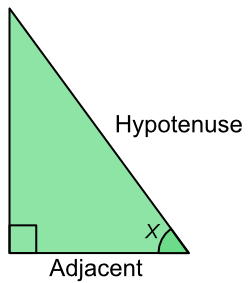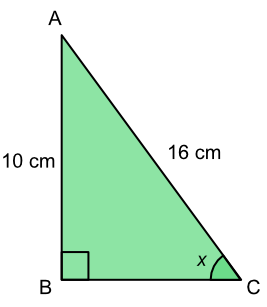Cos

## Cos

The ratio of the adjacent side to the hypotenuse is the cosine, or cos of the angle. The adjacent side is the non-hypotenuse side that touches the angle.cos (x) = frac(text(Adjacent))(text(Hypotenuse))

This is the CAH part of the mnemonic SOH CAH TOA, and gives you the formula above.

If an angle and one length (hypotenuse or adjacent) are known, the formula can be used to get the missing length. Use the cos value on your calculator to get the cos value for the angle.

If the two lengths are known, carry out the division to get the cos value for the angle. Then use the cos-1 button on your calculator to get the value for the angle.

The cos key on your calculator changes the angle value to the cos value.

The cos-1 key on your calculator changes the cos value to the angle value.

## Example 1

What is the length of BC? Give your answer to 2 decimal places.CAH Cos (x) =frac(text(Adjacent))(text(Hypotenuse)) substitute sin (36) =frac(BC)(12) find sin(36) 0.809 =frac(text(BC))(12) x12 both sides 0.809 xx 12 = BC 9.708 = BC to 2dp 9.71 = BC

What is the value of the angle x? Give your answer to 1 decimal place.CAH Cos (x) =frac(text(Adjacent))(text(Hypotenuse)) substitute cos (x) =frac(10)(16) =0.625 cos-1 both sides x =cos^-1 (0.625) = 38.68º to 1 dp = 38.7º